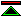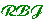# Overview:

 An introduction to some of the interesting philosophical problems which concern mathematics.
 Meaning Mathematicians use special languages for talking about strange things, out of this world. What does it all mean? Ontology What are these strange things that mathematicians talk about? Do they really exist? How can we tell? Does it matter?
 Epistemology Mathematics has often been presented as a paradigm of precision and certainty, but some writers have suggested that this is an illusion. How can we know the truth of mathematical propositions? Application How can knowledge of abstract mathematics be applied in the real world?
 Foundations Mathematics is a highly structured logical science, but dig deep enough and you can find some sand. Making the best foundations for mathematics involves philosophy. Computing What are the implications for mathematics of the information revolution? What can mathematics contribute?

# Meaning:

 Mathematicians use special languages for talking about strange things, out of this world. What does it all mean?
 God Given Does mathematical language have some pre-ordained definite meaning beyond the discretion of mortal mathematicians? If this were the case, then a Philosopher might by some means divine and articulate this meaning, helping to eliminate those numerous errors which occur throughout history because individual mathematicians miscontrue the true meaning of the concepts they are reasoning about. Culturally Evolved Alternatively we could argue that the language of mathematics is an aspect of human culture which has evolved over a long period of time, and has no independent pre-ordained meaning. To understand the meaning of mathematical language you might then study the use of that language both in the present and through history. When you understand its use, then you know the meaning of the language.
 Formally Defined If you do understand the use of the language of mathematics, you will know that mathematicians, and now logicians, sometimes take deliberate steps to sharpen their language. In recent history the use of formal notations has become significant in sharpening the less formal language of working mathematicians. In particular, the formalisation of set theory has sharpened the meaning of concepts from set theory which are then used in defining all other mathematical concepts. Formally Meaningless The introduction of formal notations has also lead some to argue that mathematical notation should be regarded as meaningless signs manipulated according to formal rules. Some constructive mathematicians undermine the importance of semantics in a different way by regarding as true just those statements which are provable.
 Designing Languages In the second half of the 20th century there has been an explosion of activity in devising formal notations, stimulated by the usefulness of such notations when working with computers. Though this has had little impact on the language of mathematicians, it may be that in the future the predominant way in which the meaning of the language of mathematics is determined is through a deliberate activity of language design, in which not only the form of the language is codified, but also its meaning or semantics. An understanding of the potential benefits using such languages, and of the techniques for undertaking such language design activities, may therefore be of interest to philosophers.
 Tarksi and Set Theory The best established account of the semantics of first order logic is that of Tarski, from which has sprung the major subdivision of Logic known as Model Theory. To the extent that mathematicians accept their mathematics as being founded in first order set theory the combination of Model theory and Set Theory provides an account of the meaning of Mathematical Propositions. Abstract Nonesense Many would now dissent from the combination of Tarski and set theory. There are the various schools of constructive mathematics which find first order set theory an unacceptable starting point for mathematics. Dissent also comes from some pure mathematicians who regard the definite prescriptions for constructing entities such as numbers or functions from sets as insufficiently abstract. Pure mathematicians may prefer to regard statements about numbers not as referring to any specific entities, but as about a particular kind of abstract structure which can be formed from many alternative constituents.

# Ontology:

 What are these strange things that mathematicians talk about? Do they really exist? How can we tell? Does it matter?
 Abstract v. Concrete Concrete Russell Bertrand Russell believed that the axiom of infinity, which states (in this context) that there are infinitely many individuals, is contingent. He believed that to discover whether there are infinitely many individuals one must observe the real world. Russell's Theory of Types can therefore be understood as speaking of concrete individuals in the real world. Abstract Zermelo By contrast, Zermelo set theory (published in the same year) is a pure set theory, in which the only things which exists are sets, themselves abstract entities, which don't even have concrete individuals as their members. It is now generally accepted that insofar as mathematics is concerned with ontology it concerns only abstract entities.
 Formal Criteria We cannot hope to discover whether abstract entities exist by observation. This creates some uncertainty about whether questions about abstract ontology are meaningful. There is therefore a temptation to fall back on formal criteria. David Hilbert asserted that the criteria for introducing some new mathematical concept were first that it should engender no contradiction, and after that simply that it should prove useful. Pragmatic Criteria Hilbert's formal criterion of consistency fails to settle ontological questions in an absolute manner, since two ontological extensions may together give rise to a contradiction even though each extension is by itself consistent. One must therefore fall back on pragmatics, and ontology becomes partly discretionary.
 Abstract Lego Arguably the role of ontology in mathematical foundations reverses Hilbert's formal criterion. For a mathematical theory to be worth investigating it must be consistent. Otherwise anything is provable and a proof has no value or content. To show a theory consistent one must exhibit a model. The role of ontology in the foundations of mathematics is to provide the building blocks and construction techniques for making models of theories to establish their consistency. This connects with the view that mathematics is essentially the logical derivation of conclusions which flow from the definitions of the mathematical concepts involved. In order to be able to define rather than axiomatise mathematical concepts you need to have the ontology in place.

# Epistemology:

 Mathematics has often been presented as a paradigm of precision and certainty, but some writers have suggested that this is an illusion. How can we know the truth of mathematical propositions?
 Logicism It is generally accepted that mathematical propositions are a priori and that they are to be established by logical proof. From here to the belief that mathematical truths are also logical truths is a small but widely contested step. Logicism, if it is accepted, reduces the epistemology of mathematics to that of logic.
 Foundations Even without full logicism a foundational view of mathematics effects an epistemological reduction. Even if set theory is considered to go beyond logic, it remains a satisfactory foundation for classical mathematics, and once the epistemological status of set theory is established that of the rest of classical mathematics follows.
 Constructive Mathematics Intuitionism rejects classical set theory, and so epistemological reduction to set theory will not do. There are such a variety of constructive approaches to mathematics that it is doubtful that any single epistemological theory could encompass them all.

# Application:

 How can knowledge of abstract mathematics be applied in the real world?
 Instantiation One way to approach the application of classical mathematics to the real world might be to introduce additional constants in the first order set theory to refer to things in the real world, and then to instantiate general mathematical theories to apply to these objects. This is not so easy to carry through. FIrst off, you have immediately changed the mathematical theory, since is no longer a pure set theory, though this is not a serious problem. Introducing a name for something doesn't do much good by itself. To apply the mathematics you have to know something about it. Since it isn't a set you can't define it and some extra axioms will be necessary.
 Abstract Models A better approach is to stay away from the real world a bit longer. In fact, stay away until you have finished doing mathematics. The way you apply mathematics to the real world is to construct an abstract model of the aspect of reality you are concerned with. This can be done by introducing appropriate definitions in set theory. Mathematics can then be applied to the analysis of this model. The results are then informally translated into conclusions about the real world.

# Foundations:

 Mathematics is a logical science, cleanly structured, and well-founded. Here we look at those foundations.
 What is a "foundation" for mathematics? We discuss some of the ways the word "foundation" is used in relation to mathematics. History of Foundations An assortment of historical cameos, starting in ancient Greece, running through the turbulent present into an exiting future.
 Logical Foundation Systems The methods of mathematics are deductive, and logic therefore has a fundamental role in the development of mathematics. Suitable logical frameworks in which mathematics can be conducted can therefore be called logical foundation systems for mathematics. Alternative Foundation Systems A bewildering variety of alternative foundation systems are superficially surveyed.

# Computing:

 What are the implications for mathematics of the information revolution? What can mathematics contribute?
 The first half of this century may be thought of as a working out in Mathematical Logic of the consequences of the fundamental advances in Logic and Mathematics made at the end of the last century, particularly those associated with Frege. In the second half of the century the development of the digital computer has become a major factor influencing the development of mathematical logic.
 The need to communicate effectively with Digital Computers has lead to an explosion in the number of formal languages in use, and in our understanding of the theory and practice of working with formal notations. While the majority of these formal notations are programming languages, the minority which are formal logics present additional problems for our understanding of the nature of Logic and Mathematics.
 As the strength and maturity of Theoretical Computer Science has grown it has become an important influence upon Logic and Mathematics.©created 1998/7/28 modified 1998/07/30